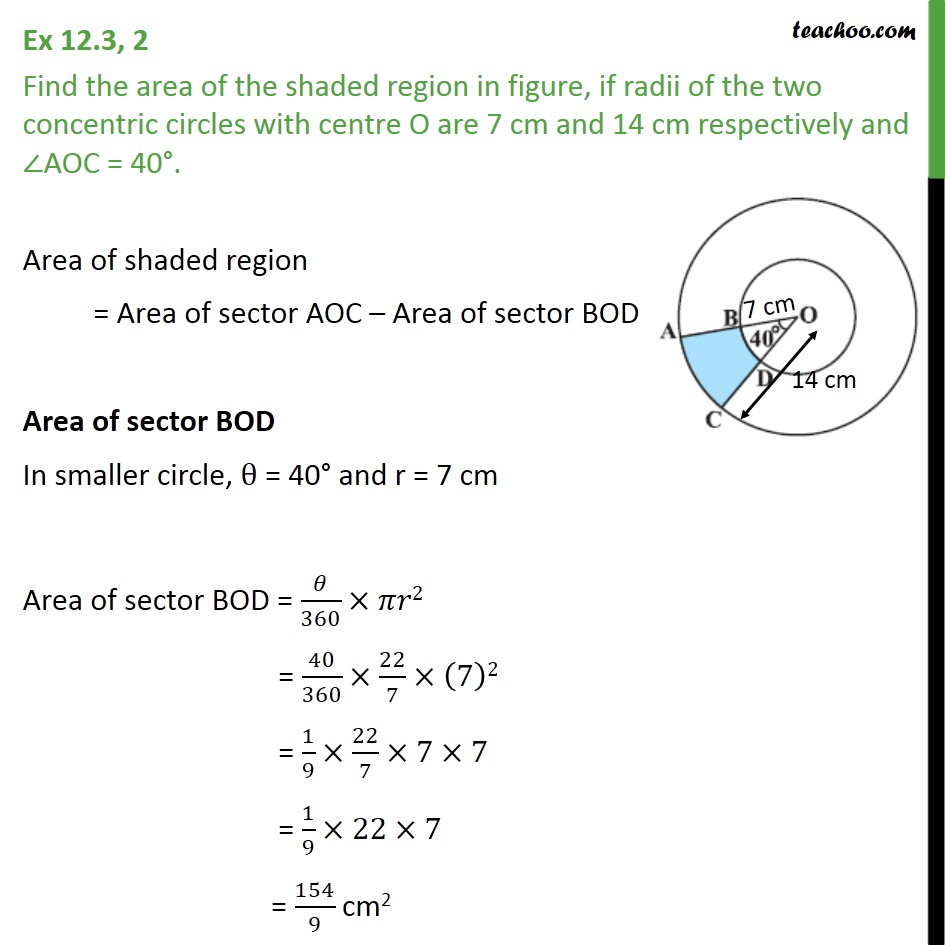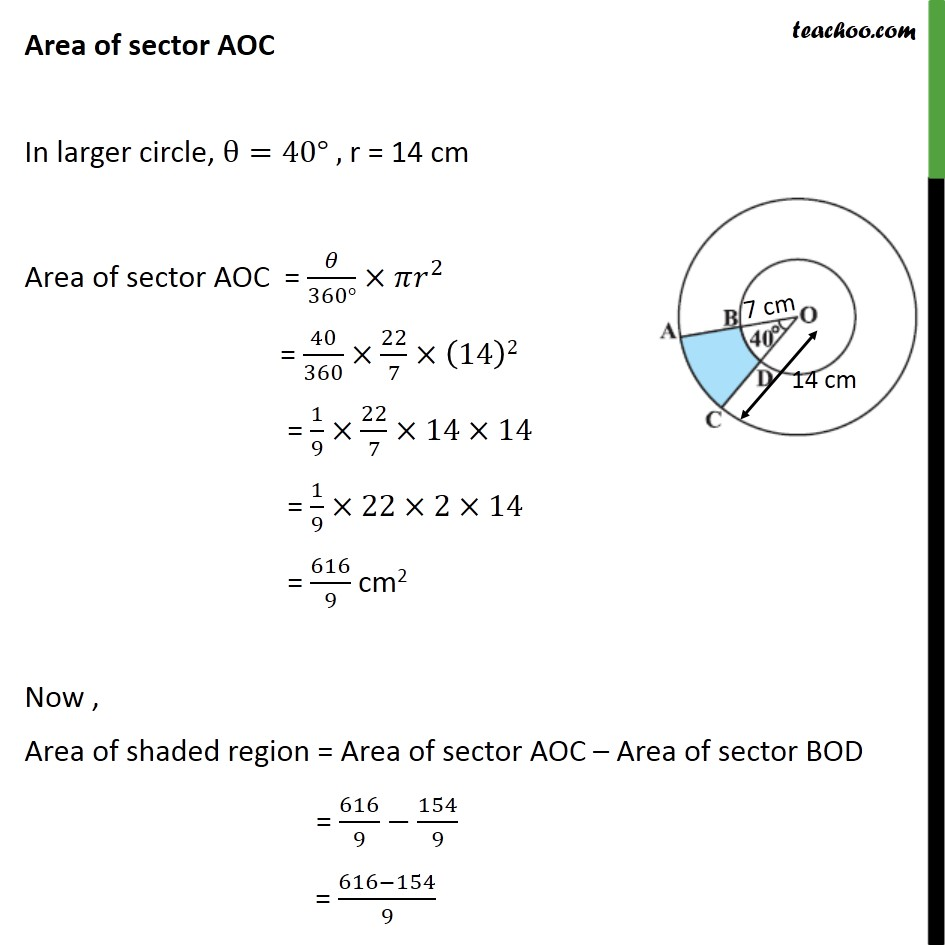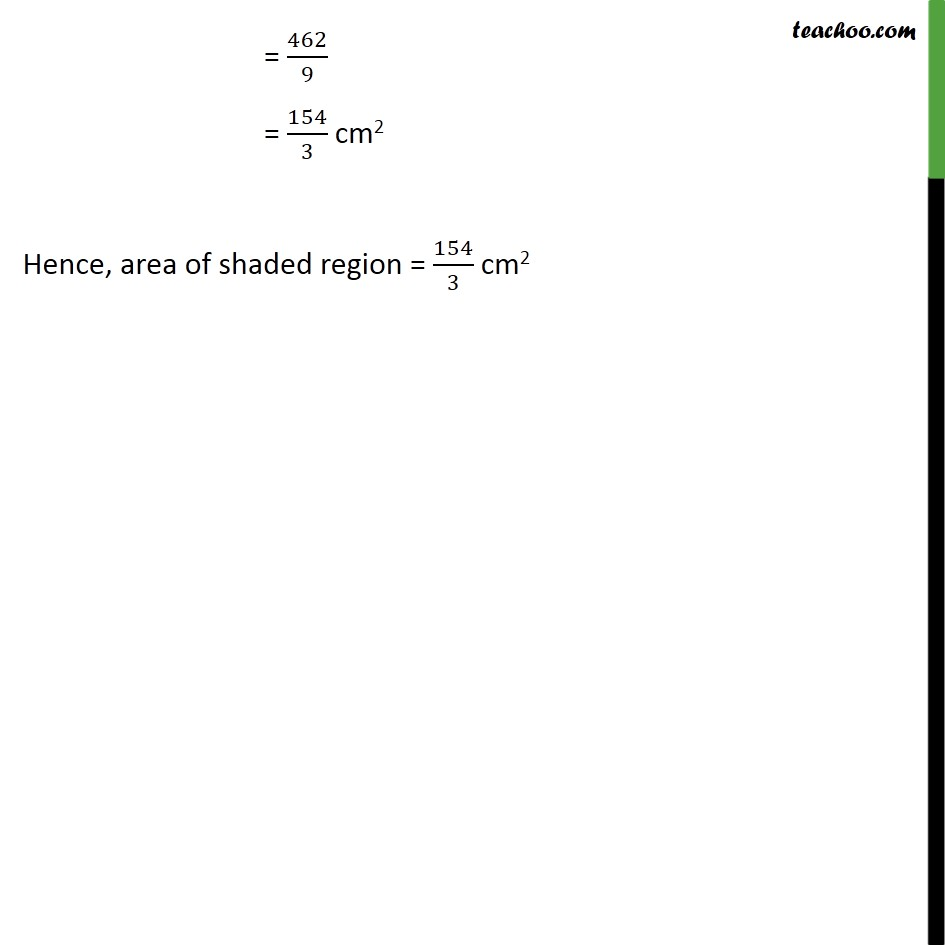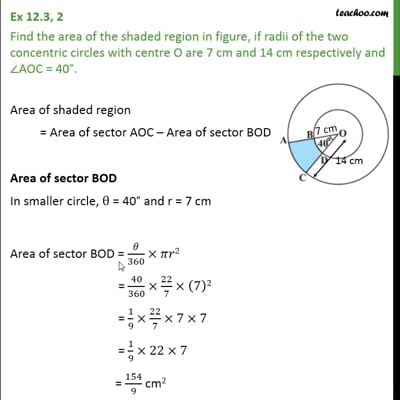Area of combination of figures : sector based

Chapter 12 Class 10 Areas related to Circles
Concept wiseThis video is only available for Teachoo black users

Introducing your new favourite teacher - Teachoo Black, at only ₹83 per month

### Transcript

Ex 12.3, 2 Find the area of the shaded region in figure, if radii of the two concentric circles with centre O are 7 cm and 14 cm respectively and ∠AOC = 40°. Area of shaded region = Area of sector AOC – Area of sector BOD Area of sector BOD In smaller circle, θ = 40° and r = 7 cm Area of sector BOD = 𝜃/360×𝜋𝑟2 = 40/360×22/7×(7)2 = 1/9×22/7×7×7 = 1/9×22×7 = 154/9 cm2 Area of sector AOC In larger circle, θ=40° , r = 14 cm Area of sector AOC = 𝜃/(360°)×𝜋𝑟^2 = 40/360×22/7×(14)2 = 1/9×22/7×14×14 = 1/9×22×2×14 = 616/9 cm2 Now , Area of shaded region = Area of sector AOC – Area of sector BOD = 616/9−154/9 = (616−154)/9 = 462/9 = 154/3 cm2 Hence, area of shaded region = 154/3 cm2